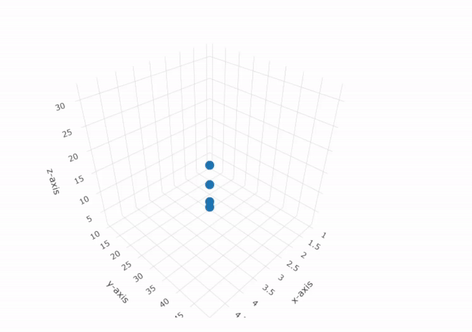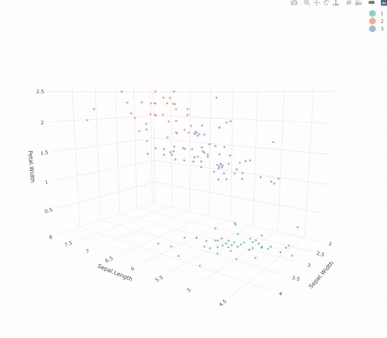Open in App
Not now

# How to Plot in 3D clusters using plotly package

• Difficulty Level : Expert
• Last Updated : 13 Feb, 2023

R-Language is widely used in Data Science, Data Visualization Data Analysis many more, etc. Plotly package is highly rich in plotting various graphs, These graphs/charts are highly interactive and user-friendly.

The 3D cluster visualizes the similarity between variables as 3-D spatial relationships. Each point in the graph represents an individual property.  the points which are closer together were more frequently sorted into the same category or class.

```Using Example:
Plotting the following dataset in 3-D plot using plotly library in R Programming Language.```

Syntax:

plot_ly(data_object, x, y, z)

where:

• data_object: Represents the dataset or dataframe object.
• x : Represent x-data vector.
• y : Represent y-data vector.
• z : Represent z-data vector.

Example 1: In this example, we will create an exemplary dataset and then plot a 3D Cluster graph using that.

## R

 `#Importing plotly library``library``(plotly)` `#Creating Dataframe``x = ``c``(1, 2, 3, 4, 5)``y = ``c``(10, 20, 30, 40, 50)``z = ``c``(2, 4, 8, 16, 32)``df = ``data.frame``(x, y, z)``df` `#Plotting 3-D Scatter plot.``#Pass dataframe and axes``plt <- ``plot_ly``(df, x = ~x, y = ~y, z = ~z)` `#Add markers to the chart``plt <- plt %>% ``add_markers``()` `#Labeling the axes.``plt <- plt %>% ``layout``(scene = ``list``(xaxis = ``list``(title = ``'x-axis'``),``                                   ``yaxis = ``list``(title = ``'y-axis'``),``                                   ``zaxis = ``list``(title = ``'z-axis'``)))``plt`

Output:Example 2: In this example, we will use the iris dataset and then plot it with the original labels using any three independent features.

## R

 `#Importing Library``library``(plotly)` `#Using iris dataset``#Removing Categorical Values``data = iris[, 1:4]` `#Finding Clusters``data\$cluster = ``factor``(``kmeans``(data, 3)\$cluster)` `#Plotting the Data``clust<- ``plot_ly``(data, x=~Sepal.Length,``                ``y=~Sepal.Width,``                ``z=~Petal.Width,``                ``color=~cluster) %>%``add_markers``(size=1.5)` `#Printing 3-D Clusters``clust`

Output:My Personal Notes arrow_drop_up